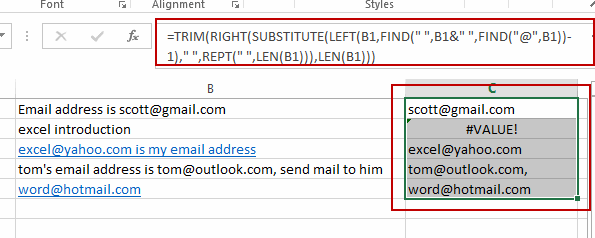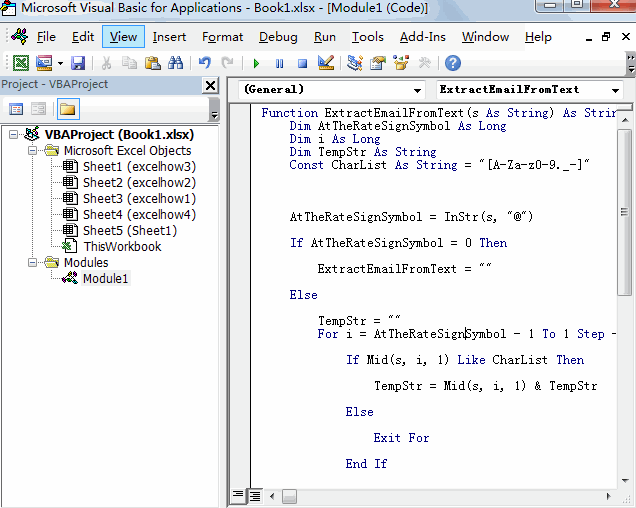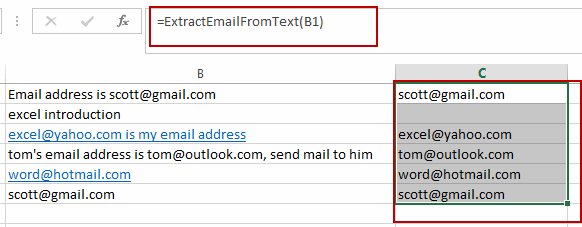# Extract Email Address from Text

This post will guide you how to extract email address from a text string in Excel. How do I use a formula to extract email address in Excel. How to extract email address from text string with VBA Macro in Excel.

Assuming that you have a list of data in range B1:B5 which contain text string and you want to extract all email addresses from those text string. How to achieve it. You can use a formula or VBA Macro to achieve the result. Let’s see the below introduction.

## Extract Email Address from Text with a Formula

To extract email address from text string in cells, you can use a formula based on the TRIM function, the RIGHT function, the SUBSTITUTE function, the LEFT function, the FIND function, the REPT function and the LEN function. Just like this:

`=TRIM(RIGHT(SUBSTITUTE(LEFT(B1,FIND(" ",B1&" ",FIND("@",B1))-1)," ",REPT(" ",LEN(B1))),LEN(B1)))`

Select the adjacent Cell C1, and type this formula, and press Enter key in your keyboard, and then drag the AutoFill Handle over other cells to apply this formula.## Extract Email Address from Text with User Defined Function

You can also write a User Defined Function to extract email address quickly, just do the following steps:

#1 open your excel workbook and then click on “Visual Basic” command under DEVELOPER Tab, or just press “ALT+F11” shortcut.#2 then the “Visual Basic Editor” window will appear.

#3 click “Insert” ->”Module” to create a new module.#4 paste the below VBA code into the code window. Then clicking “Save” button.```Function ExtractEmailFromText(s As String) As String
Dim AtTheRateSignSymbol As Long
Dim i As Long
Dim TempStr As String
Const CharList As String = "[A-Za-z0-9._-]"

AtTheRateSignSymbol = InStr(s, "@")

If AtTheRateSignSymbol = 0 Then
ExtractEmailFromText = ""
Else
TempStr = ""
For i = AtTheRateSignSymbol - 1 To 1 Step -1
If Mid(s, i, 1) Like CharList Then
TempStr = Mid(s, i, 1) & TempStr
Else
Exit For
End If
Next i
If TempStr = "" Then Exit Function
TempStr = TempStr & "@"
For i = AtTheRateSignSymbol + 1 To Len(s)
If Mid(s, i, 1) Like CharList Then
TempStr = TempStr & Mid(s, i, 1)
Else
Exit For
End If
Next i
End If
If Right(TempStr, 1) = "." Then TempStr = Left(TempStr, Len(TempStr) - 1)
ExtractEmailFromText = TempStr
End Function

```

#5 Type the following formula into blank cells and then press Enter key.

`=ExtractEmailFromText(B1)`

Type this formula into a blank cell and then press Enter key in your keyboard.

#6 lets see the result:Related Posts

How to Extract First Letter from Each Word in a Cell in Excel

This post will guide you how to extract first letter from each word in a given cell in Excel. How do I extract the first letter of each word in a range of names in your current worksheet in Microsoft ...

How to ignore Blank Cells while Concatenating cells in Excel

This post will guide you how to concatenate cells but ignore all blank cells in your worksheet in Excel. How do I concatenate cells but ignore blank cells with a formula in Excel. How to create a concatenate formula to ...

How to Calculate the Average Excluding the Smallest & Highest Numbers in Excel

Calculating the average for a batch of data is frequently used in our daily life. But for some cases like statistic the average score in a competition, or price analysis, we often calculate the average excluding the smallest and highest ...

How to Get the Maximum or Minimum Absolute Value in Excel

It is easy to find the maximum or minimum value in a batch of data in excel, but if this batch of numbers contains both positive and negative numbers, the maximum or minimum absolute value cannot be found out by ...

How to Count Only Numbers in Bold in a Range of Cells in Excel

This post will guide you how to Count only numbers with bold style in a range of cells in Excel. How do I Count on cells with bold font within a range of cells using User defined function in Excel ...

How to Sum Only Numbers in Bold in a Range of Cells in Excel

This post will guide you how to sum only numbers with bold style in a range of cells in Excel. How do I sum on cells with bold font within a range of cells using User defined function in Excel ...

How to Filter Formula in Excel

This post will guide you how to filter your data with an Formula in Excel. How do I filter cells with formulas through a User defined function in Excel 2013/2016. Filter Formula Assuming that you have a list of data ...

How to Count Cells by Font Color in Excel

This post will guide you how to count cells by font color in Excel. How do I Count the number of cells with specific cell Font color by using a User Defined function in Excel. Count Cells by Font Color ...

How to Highlight Cells Containing Formulas in Excel

This post will guide you how to highlight cells in which contain formulas using Conditional Formatting feature in Excel. How do I conditionally format a cell if it contains formula using a User defined function in combination with Conditional Formatting ...

How to Extract Number from Text String in Excel

This post will guide you how to extract number from a given test string in Excel. How do I extract all numbers from string using a formula in Excel. How to get all number from a given test string using ...

Sidebar